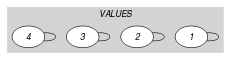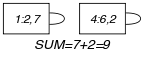## 5.392. sum_set

Origin

H. Cambazard

Constraint

$\mathrm{𝚜𝚞𝚖}_\mathrm{𝚜𝚎𝚝}\left(\mathrm{𝚂𝚅},\mathrm{𝚅𝙰𝙻𝚄𝙴𝚂},\mathrm{𝙲𝚃𝚁},\mathrm{𝚅𝙰𝚁}\right)$

Arguments
 $\mathrm{𝚂𝚅}$ $\mathrm{𝚜𝚟𝚊𝚛}$ $\mathrm{𝚅𝙰𝙻𝚄𝙴𝚂}$ $\mathrm{𝚌𝚘𝚕𝚕𝚎𝚌𝚝𝚒𝚘𝚗}\left(\mathrm{𝚟𝚊𝚕}-\mathrm{𝚒𝚗𝚝},\mathrm{𝚌𝚘𝚎𝚏}-\mathrm{𝚒𝚗𝚝}\right)$ $\mathrm{𝙲𝚃𝚁}$ $\mathrm{𝚊𝚝𝚘𝚖}$ $\mathrm{𝚅𝙰𝚁}$ $\mathrm{𝚍𝚟𝚊𝚛}$
Restrictions
 $\mathrm{𝚛𝚎𝚚𝚞𝚒𝚛𝚎𝚍}$$\left(\mathrm{𝚅𝙰𝙻𝚄𝙴𝚂},\left[\mathrm{𝚟𝚊𝚕},\mathrm{𝚌𝚘𝚎𝚏}\right]\right)$ $\mathrm{𝚍𝚒𝚜𝚝𝚒𝚗𝚌𝚝}$$\left(\mathrm{𝚅𝙰𝙻𝚄𝙴𝚂},\mathrm{𝚟𝚊𝚕}\right)$ $\mathrm{𝚅𝙰𝙻𝚄𝙴𝚂}.\mathrm{𝚌𝚘𝚎𝚏}\ge 0$ $\mathrm{𝙲𝚃𝚁}\in \left[=,\ne ,<,\ge ,>,\le \right]$
Purpose

Let $\mathrm{𝚂𝚄𝙼}$ denote the sum of the $\mathrm{𝚌𝚘𝚎𝚏}$ attributes of the $\mathrm{𝚅𝙰𝙻𝚄𝙴𝚂}$ collection for which the corresponding values $\mathrm{𝚟𝚊𝚕}$ occur in the set $\mathrm{𝚂𝚅}$. Enforce the following constraint to hold: $\mathrm{𝚂𝚄𝙼}\mathrm{𝙲𝚃𝚁}\mathrm{𝚅𝙰𝚁}$.

Example
$\left(\begin{array}{c}\left\{2,3,6\right\},\hfill \\ 〈\begin{array}{cc}\mathrm{𝚟𝚊𝚕}-2\hfill & \mathrm{𝚌𝚘𝚎𝚏}-7,\hfill \\ \mathrm{𝚟𝚊𝚕}-9\hfill & \mathrm{𝚌𝚘𝚎𝚏}-1,\hfill \\ \mathrm{𝚟𝚊𝚕}-5\hfill & \mathrm{𝚌𝚘𝚎𝚏}-7,\hfill \\ \mathrm{𝚟𝚊𝚕}-6\hfill & \mathrm{𝚌𝚘𝚎𝚏}-2\hfill \end{array}〉,=,9\hfill \end{array}\right)$

The $\mathrm{𝚜𝚞𝚖}_\mathrm{𝚜𝚎𝚝}$ constraint holds since the sum of the $\mathrm{𝚌𝚘𝚎𝚏}$ attributes $7+2$ for which the corresponding $\mathrm{𝚟𝚊𝚕}$ attribute belongs to the first argument $\mathrm{𝚂𝚅}=\left\{2,3,6\right\}$ is equal (i.e., since $\mathrm{𝙲𝚃𝚁}$ is set to $=$) to its last argument $\mathrm{𝚅𝙰𝚁}=9$.

Typical
 $|\mathrm{𝚅𝙰𝙻𝚄𝙴𝚂}|>1$ $\mathrm{𝚅𝙰𝙻𝚄𝙴𝚂}.\mathrm{𝚌𝚘𝚎𝚏}>0$ $\mathrm{𝙲𝚃𝚁}\in \left[=,<,\ge ,>,\le \right]$
Symmetry

Items of $\mathrm{𝚅𝙰𝙻𝚄𝙴𝚂}$ are permutable.

Systems
Keywords
Arc input(s)

$\mathrm{𝚅𝙰𝙻𝚄𝙴𝚂}$

Arc generator
$\mathrm{𝑆𝐸𝐿𝐹}$$↦\mathrm{𝚌𝚘𝚕𝚕𝚎𝚌𝚝𝚒𝚘𝚗}\left(\mathrm{𝚟𝚊𝚕𝚞𝚎𝚜}\right)$

Arc arity
Arc constraint(s)
$\mathrm{𝚒𝚗}_\mathrm{𝚜𝚎𝚝}$$\left(\mathrm{𝚟𝚊𝚕𝚞𝚎𝚜}.\mathrm{𝚟𝚊𝚕},\mathrm{𝚂𝚅}\right)$
Graph property(ies)
$\mathrm{𝐒𝐔𝐌}$$\left(\mathrm{𝚅𝙰𝙻𝚄𝙴𝚂},\mathrm{𝚌𝚘𝚎𝚏}\right)\mathrm{𝙲𝚃𝚁}\mathrm{𝚅𝙰𝚁}$

Graph model

Parts (A) and (B) of Figure 5.392.1 respectively show the initial and final graph associated with the Example slot.

##### Figure 5.392.1. Initial and final graph of the $\mathrm{𝚜𝚞𝚖}_\mathrm{𝚜𝚎𝚝}$ constraint(a) (b)